# GRE Subject Test: Math : Absolute Value Inequalities

## Example Questions

← Previous 1 2 Next →

### Example Question #11 : Absolute Value Inequalities

A type of cell phone must be less than 9 ounces with a tolerance of 0.4 ounces. Which of the following inequalities can be used to assess which cell phones are tolerable? (w refers to the weight).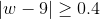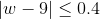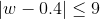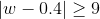Explanation:The Absolute Value Inequality that can assess which cell phones are tolerable is:### Example Question #12 : Absolute Value Inequalities

Solve for x: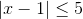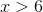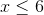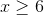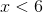Explanation:

Step 1: Separate the equation into two equations:

First Equation: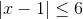Second Equation: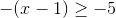Step 2: Solve the first equation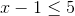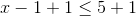Step 3: Solve the second equation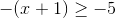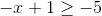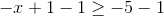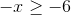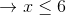The solution is← Previous 1 2 Next →

### All GRE Subject Test: Math Resources